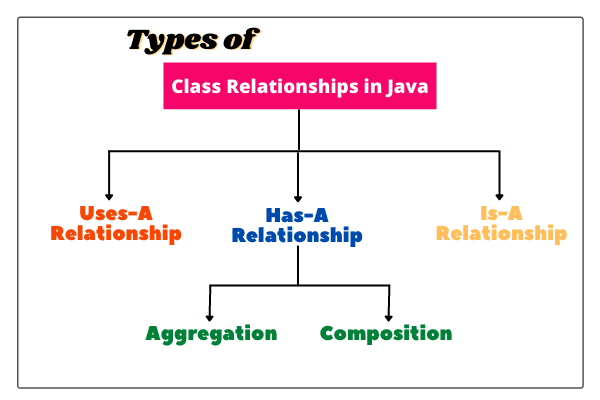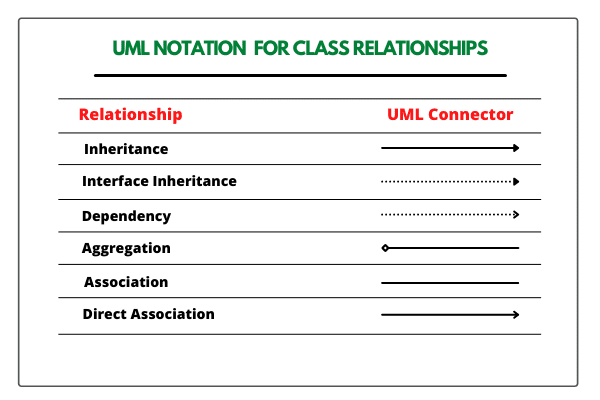# Class Relationships in Java | Types of Relationships

Class relationships in Java define the special relationships among different kinds of classes.

For example, there is a special relationship between a class named Vehicle and a class Car: A Car is a type of Vehicle.

There is a different kind of relationship among classes Shape, Circle, Rectangle, and Square. A Circle is a type of Shape. A Rectangle is a type of Shape.

When we design a major application or program, we need to explore the relationships among classes. It helps us in a number of ways.

For example, suppose in an application, we have classes with common behaviors (methods) then we can save effort by placing the common behaviors (methods) within the superclass.

Suppose some classes are not related to each other, then we can assign different programmers to implement each of them, without worrying that one of them will have to wait for the other.

For this purpose, we need to learn different types of relationships among classes in Java. Relationships among classes help to understand how objects in a program work together and communicate with each other.

## Types of Relationship among Classes in Java

There are three most common relationships among classes in Java that are as follows:

a. Dependence (“Uses-A”)
b. Association (“Has-A”)
c. Inheritance (“Is-A”)Association is further classified into aggregation and composition that will be understood in the further tutorial in detail.

Let’s understand these three relationships among classes one by one.

## Dependence (Uses-A) Relationship in Java

When we create an object of a class inside a method of another class, this relationship is called dependence relationship in Java, or simply Uses-A relationship.

In other words, when a method of a class uses an object of another class, it is called dependency in java. It is the most obvious and most general relationship in java.

Look at the below figure where a method display() of class B uses an object of class A. So, we can say that class A depends on another class B if it uses an object of class A.If several classes of an application program depend on each other, then we say that the coupling between classes is high.

It is a good programming practice to minimize the dependency between classes (i.e., coupling) because too many dependencies make an application program difficult to manage.

On the other hand, if there are few dependencies between classes, then we say that the coupling between classes is low.

## Why does coupling matter?

If a class changes its behavior in the next release of the application program, all the classes that depend on it may also be affected. In this situation, we will need to update all the coupled classes.

Therefore, if the coupling between classes will be low, we can easily manage them. Thus, we must remove unnecessary coupling between classes.

## Association (“Has-A”) Relationship in Java

Association is another fundamental relationship between classes that is informally known as “Has-A” relationship.

When an object of one class is created as data member inside another class, it is called association relationship in java or simply Has-A relationship.

Look at the above figure where an object of class A is created as data member inside another class B. This relationship is known as Has-A relationship. It is easy to understand and makes a stronger form of dependency.

We will learn Has-A relationship in more detail in the next tutorial.

## Inheritance (“Is-A”) Relationship in Java

Inheritance represents Is-a relationship in Java. It establishes a relationship between a more general class (known as superclass) and a more specialized class (known as subclass).

In other words, Is-A relationship defines the relationship between two classes in which one class extends another class.

Look at the above figure where a class B makes a relationship with class A by the keyword “extends” and can inherit data members from class A.

For more detail, go to this tutorial: Inheritance in Java | Example, Use, Advantage

## UML Notation in Java

UML stands for Unified Modeling Language. It is an international standard notation. Many Programmers use this notation to draw classes diagram that explains the relationship between classes.There is a number of tools available for drawing UML diagrams. A table in the below figure shows the UML notation for class relationships in Java.

Hope that this tutorial has covered almost all the important points related to types of class relationships in Java with the following diagrams. I hope that you will have understood this simple topic.